# Test Data

## Overview of Tests

Major portions of this section were adopted from Lizal et al. (2015). The positron emission tomography (PET) method provides the best spatial resolution (among radiological methods). In addition to local deposition in the various sections, the deposition hot spots can also be evaluated. However, in comparison to the PET methodology, which is routinely applied to clinical examination, using this method in the in vitro design requires major modiﬁcations both in the aerosol preparation and, in particular, in the experiment evaluation approach. The method, based on PET and fulﬁlling the above mentioned criteria, is presented in the following.

## The aerosol exposure procedure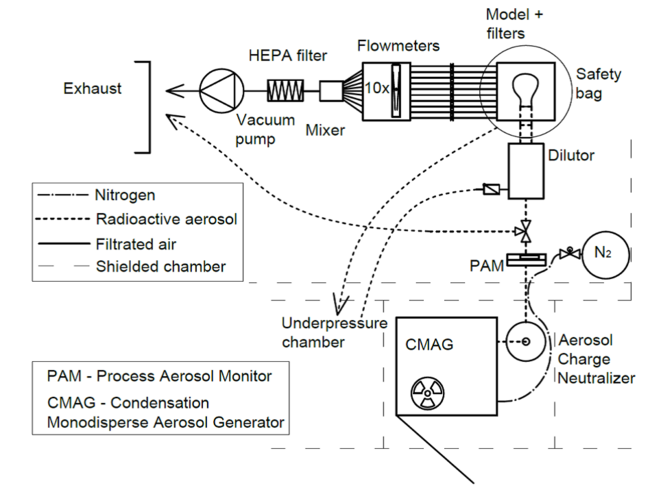Figure 5: A scheme of the experimental setup during the PET measurement of aerosol deposition.

The generated aerosol was fed through a 85Kr based NEKR-10 charge equilibrator (Eckert & Ziegler Cesio) to a PAM aerosol monitor (TSI 3375) for continuous particle size and concentration measurement. The operation and precision of the aerosol monitor was validated using Aerodynamic particle sizer (APS) TSI 3321. The validation was performed prior to the experiment using the identical setup, apart from adding of the radioactive substance into the atomizer. The size of the particles generated by CMAG was adjusted according to APS and the size displayed by the aerosol monitor was recorded. Subsequently, during the experiment, only the radioactive substance was added to the atomizer and particles of the same size were produced. Usually only a small correction of saturator ﬂow was needed at the beginning of the experiments. Only one size of particles was measured during one day; therefore no further adjustments of the generator were needed. The aerosol monitor served as an on-line indication of the process of aerosol generation being stable. It was easily accomplished, as the exposure of the model to the aerosol lasted only 5 to 15 mins. Filters consisting of Millipore AP40 glass ﬁbers were attached to the output branches of the respiratory tract model. The entire system (Figure 6) was enclosed in a plastic bag which was kept in a vacuum to prevent the active aerosol from leaking into the laboratory. All the 10 terminal branches with ﬂow meters for ﬂow rate control were combined into one branch with a protective High efﬁciency particulate air (HEPA) ﬁlter. The vacuum was generated with a Busch R, 5 PA 0008 C rotary oil vacuum pump. The ﬂow distribution in each section of the model is provided in Table 3.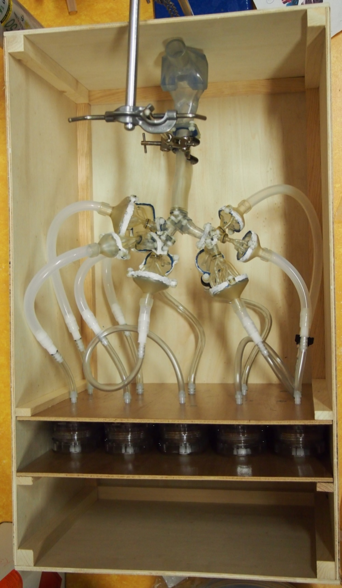Figure 6: A photograph of the physical model prior to the PET measurement.

The whole exposure of the model to the aerosol was performed in a shielded laboratory with an underpressure ventilating system, which would prevent the aerosol from escaping the room in case of a primary safety system failure. The laboratory personnel were not present in the laboratory during the exposure, with the exception of the regular instrument supervision. Whenever they had to enter the lab, they wore half mask respirators. The experiments were performed in a steady-state inhalation mode with the ﬂow rates of 15, 30, and 60 L/min. Liquid monodisperse particles with mass median aerodynamic diameter of 2.5 and 4.3${\mu m}$were used. The standard geometric deviation of size was less than 1.24 for all measured regimes. The models were exposed for 10 to 15 minutes depending on radioactivity decrease by radionuclide decay. The peak activity in the models was 4 to 60 ${Bq/cm^{2}}$(depending on the particle size and concentration, measuring mode, and model used), as measured with an RP-2000 portable contamination meter (VF Zilina, CZ).

## Deposited activity evaluation method

The model was transported to a Siemens Biograph 64 Truepoint PET-CT scanner immediately after the radioactive exposure. The transportation took approximately 3 minutes. The PET-CT scanner acquired ﬁrstly CT images, which were promptly followed by PET images.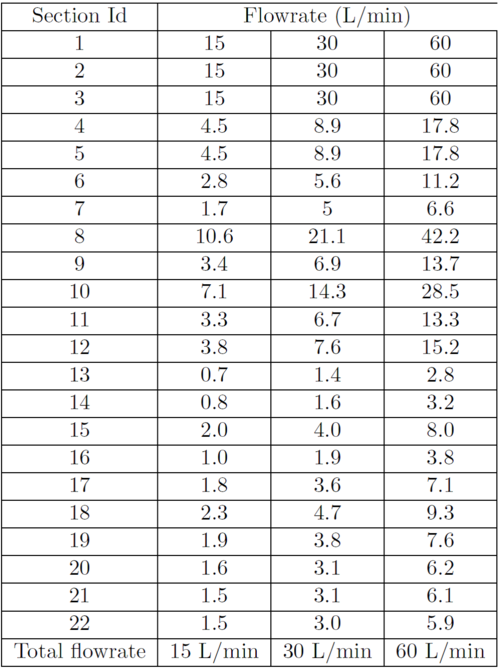Table 3: Flowrates through the individual sections of the model (section identiﬁcation numbers are presented in Figure 4). Sections 13 – 22 lead to the 10 outlets. Flowrates in the intermediated sections (4 – 12) are calculated using mass continuity.

Both the CT and PET images could be attributed unambiguously to the given geometry, owing to the assigned geometrical coordinates. The CT images are important for a precise localization of the edges of the sections, whereas the PET images contain the essential information about aerosol deposition. The CT and PET images were imported into Carimas 2.47 ver. 2.2.44.7002 SW (Turku PET Centre 2012) with the CT images as the main images and the PET images as background images. The software Carimas is a medical image processing tool, which was developed primarily for analysis of PET images at Turku PET Centre in Finland and is available as a freeware for non-commercial use from: http://www.turkupetcentre.ﬁ/carimas/download/. The Carimas was developed in an “interactive data language” (IDL) and runs on IDL Virtual Machine™, a cross-platform utility for running IDL code. It supports multiple input formats (DICOM, ECAT, Analyse, Interﬁle, Nifti, Interﬁle, MicroPET) and general bitmap formats (JPG, TIFF, PNG and BMP). Researchers can perform visualization, segmentation, statistical analysis, or modelling of PET data. It is possible to perform the image fusion, i.e. to coregistrate PET and CT or MR, images. Users can deﬁne a volume of interest (VOI) and, by using the static image analysis, they can calculate the mean activity in the VOI (in units of the PET image), standard deviation, minimal and maximal values, the number of voxels analysed, and the volume of the region. The software was tested and compared to commercial tools with an excellent agreement as documented by Nesterov et al. (2012) and Harms et al. (2014). To facilitate the identiﬁcation of the hot spots in the Carimas software, the BGRY colour system was used to display the PET images and the system range was reduced so that the hot spots were clearly seen in the model sections (Figure 7). The sections of the model were marked as volumes of interest (VOI), and the mean volume activity (in Bq/mL) was evaluated using the Carimas software. Each section was enveloped in an independent VOI. A cylinder or a sphere was selected as the starting shape of the VOI, depending on the shape of the sections. Firstly, the starting shape of the VOI was positioned and shaped as a whole to attain a suitable orientation and an adequate size. In the next step, each VOI was shaped in the vertex mode for a local mesh adjustment, so that the VOI surrounded the section with an overreach, while preventing an overlap of different VOIs. If the latter was not avoided, the observed activity would be attributed to both VOIs, resulting in biased results. The degree of mesh density can be modiﬁed by reducing the number of nodes or by enlarging or shrinking the mesh.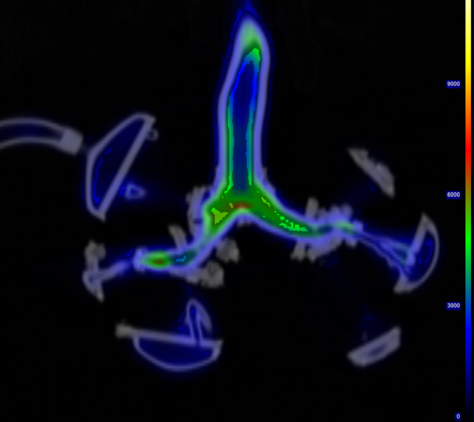Figure 7: CT and PET image presentation in the Carimas software.

With regard to the PET precision, a VOI should optimally overreach the section by roughly 5mm on all sides to cover all the radiation emitted from the section. However, due to the complex lung geometry, it was impossible to form all the VOIs with such a large overreach, and so the uncounted radioactivity was accounted for in a correction VOI. Two additional VOIs had to be created to determine the magnitude of the correction: a VOI for the top part of the box, accommodating the model with the hoses, and a correction VOI for the bottom part of the box, accommodating the ﬁlters downstream of the terminal branches. They encompass the large top space with the model and the bottom space between the two horizontal partitions respectively in Figure 6. Two separate corrections were needed for the model and ﬁlters, because the difference in volume radioactivity is within few orders of magnitude and therefore it is necessary to assign the uncounted radioactivity to its real source. It is essential to preserve the true ratio of the radiation deposited in model sections and on ﬁlters. The correction factor for the sections was calculated as the ratio of the total activity obtained from the VOI encompassing the whole model to the activity obtained by summing up the section VOIs. Similarly, the correction factor for the ﬁlters was calculated as the ratio of the total activity of the large VOI encompassing all the ﬁlters, to the sum of the activities of the ﬁlter VOIs. The corrections for the model sections (${Corr_{m}}$) and for the ﬁlters (${Corr_{f}}$) were calculated as follows:

 ${Corr_{m}={\frac {A_{cm}}{\sum _{1}^{n}A_{i}}}}$${(1)}$${Corr_{f}={\frac {A_{cf}}{\sum _{1}^{n}A_{j}}}}$${(2)}$where ${A_{i}}$and ${A_{j}}$are the activities measured in the model sections and on the ﬁlters respectively, and ${A_{cm}}$and ${A_{cf}}$are the activities measured in the correction VOI for the model and ﬁlters respectively. We assume that the distribution of uncounted radioactivity is proportional to the measured activity in the sections of the model. The higher the activity measured in the ﬁlter, the higher the activity spread around the section. Therefore, the uncounted activity is to be accounted for by the sections proportionally to their measured activity. The correction formula then is simply:

 ${A_{i}^{\mbox{*}}=Corr_{m}\cdot A_{i}}$${(3)}$${A_{j}^{\mbox{*}}=Corr_{f}\cdot A_{j}}$${(4)}$The asterisk (${\mbox{*}}$) indicates that the data has been corrected. The magnitude of the correction factor can also be regarded as a model tightness indicator. If the model was not tight enough and some aerosol leaked beyond the model, the amount of aerosol in the correction VOI would be appreciably higher than in the sections; also, the calculated correction factor would be higher. The correction factors for the model sections and for the ﬁlters lie largely within the ranges of 1.0 to 1.2 and 1.0 to 1.1 respectively. Aerosol leaks should be suspected if these ranges were exceeded. The VOIs created can be saved in VRML format in the Carimas software and imported to another model. Therefore, the same set of VOIs was used for the analysis of all models. The corrected activities served to calculate the deposition fractions (DF) for two aerosol particle sizes (DF is deﬁned as the ratio of inhaled particles deposited in the lungs section ${A_{i}^{\mbox{*}}}$to the total number of particles entering the lungs). The total amount of aerosol was calculated as the sum of activities in the model, in the output sections, in the connection hoses and on the ﬁlters. The observed activity is assumed to be directly proportional to the number of deposited particles. This is based on the fact that the radioactive atoms are dispersed uniformly in the constantly stirred atomizer and that each particle generated contains a nucleus of the same size, on which DEHS has condensed.

 ${DF_{i}={\frac {A_{i}^{\mbox{*}}}{\sum _{i}^{n}A_{i}^{\mbox{*}}}}}$${(5)}$where ${n}$is the total number of all sections including the hoses and ﬁlters.

## Measurement errors

### Sources of error in PET imaging

The quality of the images obtained with PET is affected by the following parameters: the spatial resolution, sensitivity, noise, scattered radiation and contrast. The parameters are interrelated, thus any efforts to reduce the effect of one parameter often result in an increased effect of another parameter (Saha, 2010). The accuracy of determining of the deposition point for a speciﬁc particle is related to the attainable spatial resolution of the PET scanner. The resolution is affected by the detector size, positron range, noncolinearity, reconstruction method and localization of the detectors: The actual spatial resolution of a particular PET scanner is measured by the standardized method recommended by the NEMA – National Electrical Manufacturers Association (2001). The full width at half maximum (FWHM) is the quantity serving as the measure of resolution. This quantity can be understood as the magnitude of point source “blurring” in the photograph or as the shortest distance at which two point sources can be discerned as such. The spatial resolutions (based on NEMA (2001)) for the PET Siemens Biograph 64 system are listed in Table 4.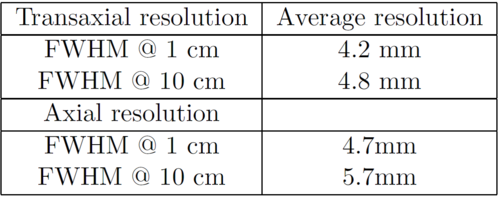Table 4: Spatial resolution of the PET scanner used for experiments (Siemens 2014)

The remaining parameters of our PET scanner were: the sensitivity 4.2 cps/kBq, noise equivalent count rate 96 kcps at 35 kBq/cc, and scatter fraction < 38% (Lizal et al., 2015). As noted in the model limitations section the possible electrical charge on the walls of the physical model should be considered. The amount of depositing particles due to the mirror charge is probably low, however, it should be conﬁrmed by an additional experiment.

### Inter-person variability

To assess the error connected to the analysis of images, in particular to the deﬁnition of VOIs, the whole process, beginning with forming the VOIs, was performed by two technicians independently and their results were compared. The average of the differences between corresponding sections was 3% of the average value of the calculated radioactivity. More experiments should be performed to calculate the actual uncertainty of the whole method, but the above mentioned test conﬁrmed that even if the VOIs were formed independently by two different individuals, their results were in close agreement (Lizal et al., 2015).

### Validation of the method

The experimental setup and deposition measuring technique was validated by optical microscopy. Nickel coated particles (M-18-Ni, Cospheric, Santa Barbara, CA, USA) with MMAD 14.1μm were dispersed by the Small Scale Powder Disperser TSI 3433 and deposited in the model. The model was disassembled and particles were rinsed out of each section using ultrasonic bath. The particles were then ﬁltered out and counted using optical microscopy. The results were consistent with the results acquired by PET. However, the presented PET method is capable of achieving a signiﬁcantly higher spatial resolution. Therefore, more detailed data on local deposition can be obtained, as the size of VOIs can be smaller than the size of the sections of the model and is limited only by the spatial resolution of the PET scanner, in our case 5 mm (Lizal et al., 2015).

## Measured data

The proposed method was used to evaluate deposition during steady-state inhalation for the following cases:

1. 15 L/min, ${d_{p}=4.3{\mu }m^{2}}$2. 30 L/min, ${d_{p}=2.5{\mu }m^{2}}$3. 60 L/min, ${d_{p}=4.3{\mu }m^{2}}$The measurement for 60 L/min was made in duplicate. The Deposition Fraction difference between the two measurements was calculated for each section, and the observed difference within the repeated measurements was drawn in the plot in the form of error lines as the estimate of measurement variability.

The plot in ﬁg. 8 demonstrates that the majority of particles was deposited in the oral cavity and in the trachea in all the applied variants. A comparison of the regimes shows that the amount of deposited particles increases with their increasing momentum, which conﬁrms that inertial impaction was the dominant deposition mechanism in our case. The results demonstrate that the in vitro deposition measurement method based on the PET technique enables the DF to be evaluated with adequate precision for distinguishing the effects of the particle size and the breathing regime.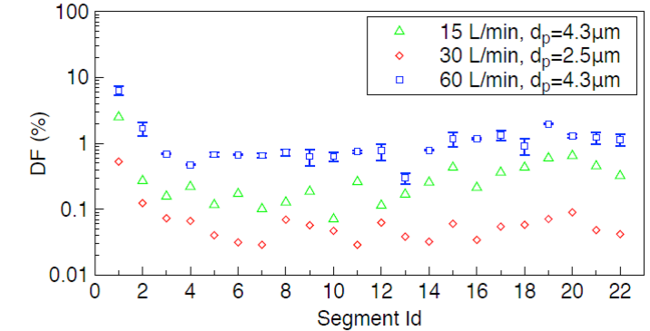Figure 8: Results obtained by measurements in the realistic model (section identiﬁcation numbers are presented in Figure 4). Error bars indicate the difference between two measurements performed on two separate, but geometrically identical models. Measurements for 15 and 30 L/min were performed just once.

In the following step, a comparison with previously published data was performed. Few studies presenting in vitro measured local aerosol deposition in tracheobronchial airways have been published in past years. The most suitable for comparison with our data were the results acquired by Zhou & Cheng (2005) and Chan & Lippmann (1980) as they both used realistic lung casts. However, their models had less generations of branching (four and six, respectively) compared to our model. The comparison of deposition efﬁciency (DE) deﬁned as the ratio of the number of particles deposited in the section to the number of particles entering the section showed close agreement with both studies mentioned above (see Figure 9). The practical calculation of DE was performed as a ratio of corrected activity in the examined section to the sum of activities in all downstream sections including ﬁlters and hoses, and the activity in the examined section:

 ${DE_{i}={\frac {A_{i}^{\mbox{*}}}{A_{f}^{\mbox{*}}+A_{i}^{\mbox{*}}}}}$${(6)}$where ${A_{f}^{\mbox{*}}}$is the corrected activity in the section downstream the i-th section. DE was plotted as a function of Stokes number deﬁned as:

 ${Stk={\frac {\rho d_{p}^{2}U}{18\mu D}}}$${(7)}$where ${d_{p}(\mu m)}$is the aerodynamic diameter of a particle, ${U(m/s)}$is the velocity, ${\mu (Pa.s)}$is the Viscosity of air and ${D(m)}$is the characteristic diameter (of the airway), which was measured in the digital geometry in Rhinoceros 4.0 software (McNeel Seattle, WA7 USA). The diameter of the entrance airway was used as the characteristic diameter, in accordance with Zhou & Cheng (2005). Chan & Lippmann (1980) introduced the following correlation ﬁt based on their experimental data for the ﬁrst six generations:

 ${DE=1.606Stk+0.0023}$${(8)}$Similar correlation ﬁt was calculated based on the current data for 1st to 4th generation.

 ${DE=1.038Stk+0.0012}$${(9)}$Both the empirical equations were plotted in the graph of Figure 9. The airways in the 4th to 7th generation, which create separate parts of the model (identiﬁcation numbers 13 to 22), were evaluated as a whole section and plotted in Figure 9 as well. The deposition fraction can be calculated in a higher resolution (creating smaller sections) down to the resolution of the PET scanner (5Â mm). This resolution is signiﬁcantly better than the resolution of other commonly used methods (e.g. gravimetry or ﬂuorometry), but the evaluation of deposition in single airways with diameters smaller than PET scanner resolution is not possible.

The measured Deposition data reported in ﬁgure 8 can be found in ﬁle DF_in-vitro.xlsx.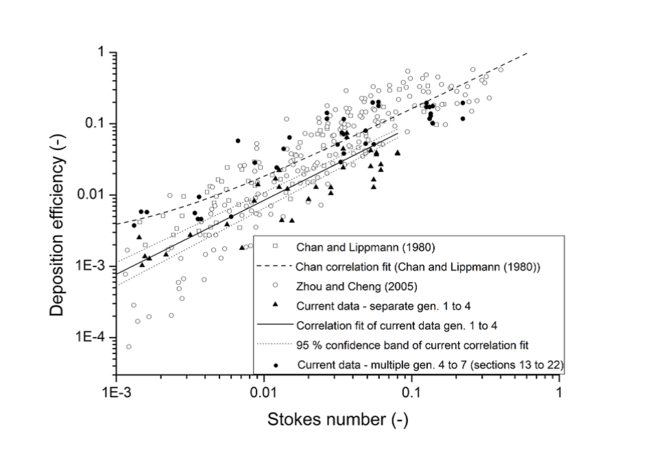Figure 9: The comparison of the current results with previously published experimental data (symbols) and correlation ﬁts (lines). The ﬁlled triangles represent the current data for single bifurcations in the 1st to 4th generation. The ﬁlled circles represent the current data for the model sections no. 13 to 22 (Figure 4), which contain multiple bifurcations.

Contributed by: P. Koullapis, F. Lizal, J. Jedelsky, L. Nicolaou, K. Bauer, O. Sgrott, M. Jicha, M. Sommerfeld, S. C. Kassinos — University of Cyprus